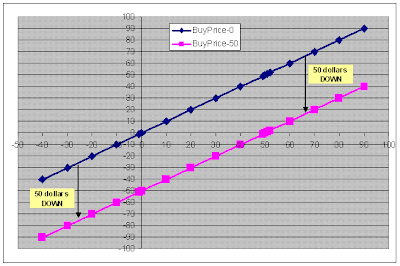Continuing further from the 3rd part Options Payoff Functions Introduction, here is the 4th part of the series on option trading tutorials. We’ve seen how to plot the graphs and look at profit and loss for our investment in stocks, as the stock price fluctuates.

Remember the first graph, which was introduced with a ZERO buy Price? Here it is:Then, remember the 2nd graph where we assumed a buy price of 50 dollars? Here it is:Now suppose we draw these 2 graphs on the same sheet, and extend the price ranges, we get the following:.
Let's head over to the next part Payoff Functions Summary & Conclusions
 Posted by Shobhit See All Articles with